Courses

# Test: Thermodynamics And Thermochemistry- 2

## 30 Questions MCQ Test Physical Chemistry | Test: Thermodynamics And Thermochemistry- 2

Description
This mock test of Test: Thermodynamics And Thermochemistry- 2 for Chemistry helps you for every Chemistry entrance exam. This contains 30 Multiple Choice Questions for Chemistry Test: Thermodynamics And Thermochemistry- 2 (mcq) to study with solutions a complete question bank. The solved questions answers in this Test: Thermodynamics And Thermochemistry- 2 quiz give you a good mix of easy questions and tough questions. Chemistry students definitely take this Test: Thermodynamics And Thermochemistry- 2 exercise for a better result in the exam. You can find other Test: Thermodynamics And Thermochemistry- 2 extra questions, long questions & short questions for Chemistry on EduRev as well by searching above.
QUESTION: 1

Solution:
QUESTION: 2

Solution:
QUESTION: 3

### Consider the reaction: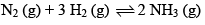Carried out at constant temperature and pressure. If ΔH and ΔU are enthalpy and internal energy changes for the reaction, which of the following expressions is true?

Solution: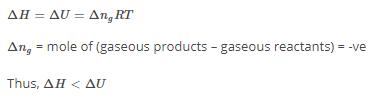QUESTION: 4

The work done in ergs for the reversible expansion of one mole of an ideal gas from a volume of 10L to 20L at 250C is:

Solution:
QUESTION: 5

Work done during isothermal expansion of 1 mole of an ideal gas from 10 atm to 1 atm at 300K is:

Solution:
QUESTION: 6

ΔE° of combustion of isobut ylene is -x KJ mol-1. The value of ΔH° is:

Solution:
QUESTION: 7

The free energy change for a reversible reaction at equilibrium is:

Solution:
QUESTION: 8

The heat of neutralization of a strong acid and a strong alkali is 57.0 KJ mol–1. The heat released when 0.5 mole of HNO3 solution is mixed with 0.2 mole of KOH is:

Solution:
QUESTION: 9

Heat of formation of H2O is –188 KJ per mole and H2O2 is –286 KJ mol–1. The enthalpy change for the reaction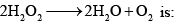Solution:

ΔHreaction​=(∑Hproducts​)−(∑Hreactants​)

Δ Hreaction=[(ΔHO2​​)+(ΔHH2​O​)]−[ΔHH2​O2​​]

ΔHO2​​=0,ΔHH2​O​=−286KJ per mole, ΔHH2​O2​​=−188KJ per mole

put the values ,

ΔH{reaction}=-196 KJ per mole

QUESTION: 10

The species which by definition has ZERO standard molar enthalpy of formation at 298 K is

Solution:
QUESTION: 11

Given that bond energies of H–H and Cl–Cl are 430 and 240 KJ mol–1 respectively, and ΔHf for HCl is -90 KJ mol-1. Bond enthalpy of HCl is:

Solution:
QUESTION: 12

Hess’s Law deals with:

Solution:
QUESTION: 13

One mole of methanol when burnt in O2 gives out 723 KJ mol–1 heat. If one mole of O2 is used, what will be the amount of heat observed:

Solution:

(1) CH3OH +3232O2→→CO2 + 2H2O, ΔH∆H=-723 kJ

The heat evolved for one mole of oxygen = -723x2/3 = -482 kJ

QUESTION: 14

The enthalpy and entropy change for the reaction: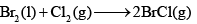Are 30 KJ mol–1 and 105 JK–1mol–1 respect ively. The temperature at which the reaction will be in equilibrium is:

Solution:
QUESTION: 15

The change in the entropy for the fusion of 1 mole of ice is:

(Melting point of ice = 273 K, molar enthalpy of fusion of ice = 6.0 KJ mol–1):

Solution:

Change in Entropy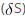=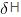(Enthaply of fusion) /T (Temperature)

= 60*10/ 273

= 21.97 J/K/mol

QUESTION: 16

For a spontaneous process, the correct statement is:

Solution:
QUESTION: 17

The abso lute enthalpy of neutralization of the reaction: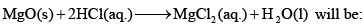Solution:
QUESTION: 18

For the reaction of one mole of zinc dust with one mole of sulphuric acid in a bomb calorimeter, ΔU and W corresponds to:

Solution:
QUESTION: 19

Standard enthalpy and standard entropy changes for the oxidation of NH3 at 298 K are –382.64 KJ mol–1 and – 145.6 JK–1mol–1, respectively. Standard Gibb’s energy change for the same reaction at 298 K is:

Solution:
QUESTION: 20

ΔG = ΔH-TΔS was given by:

Solution:
QUESTION: 21

The heat capacity of 10 mol of an ideal gas at a certain temperature is 300 JK–1 at constant pressure. The heat capacity of the same gas at the same temperature and at constant volume would be:

Solution:
QUESTION: 22

The internal pressure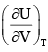of a real gas is related to the compressibility factor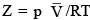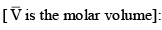Solution:
QUESTION: 23

Entropy is:

(I) It is the degree of disorder of randomness in a system
(II) Entropy is a state function and mixing is always positive
(III) Entropy is a intensive property
(IV) Entropy of solid is minimum and gases is maximum
(V) Entropy increase wit h temperature, complexity and atomicity

The correct statement is:

Solution:
QUESTION: 24

From the following pairs, choose the substances with the higher entropy per mole at a given temperature:

(I) O2 gas at 5 atm or O2 gas at 0.5 atm
(II) Br2 (L) or Br2 (g)

Solution:
QUESTION: 25

For the gaseous substance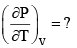Solution:
QUESTION: 26

Among the following, the system require higher amount of thermal energy to giving the temperature 800C:

Solution:
QUESTION: 27

What is the internal pressure for 4 mole of Vander Waals gas:

Solution:
QUESTION: 28

Which relation is correct in this equation: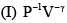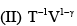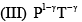Solution:
QUESTION: 29

Which of the following statement is correct: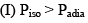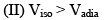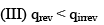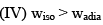Solution:
QUESTION: 30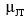for ideal and Vander Waals gases respectively:

Solution: